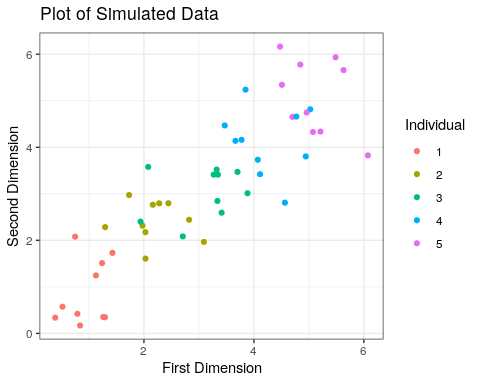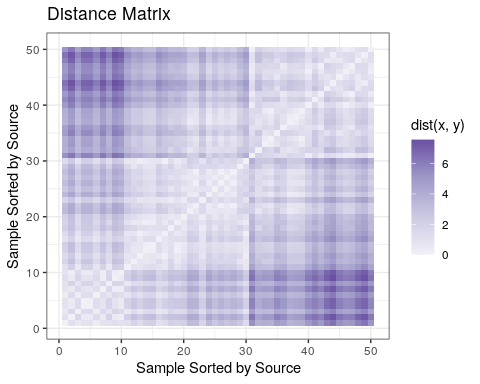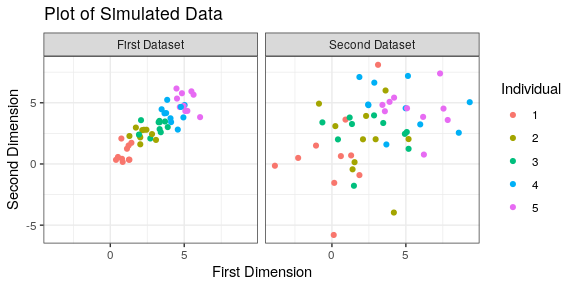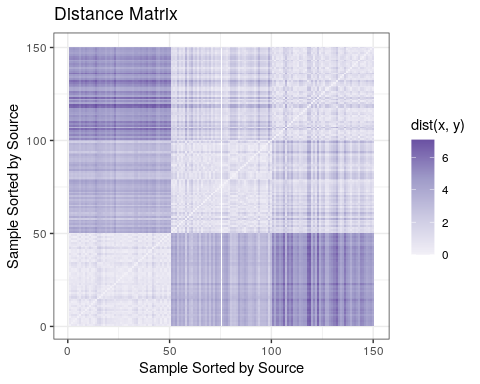Discriminability Statistic

In this notebook, we will discuss the basics of the discriminability statistic in a real dat simulated and real data context, and demonstrate some of the useful plots that users may want to visualize the results with.

library(mgc)
library(reshape2)
library(ggplot2)

plot_mtx <- function(Dx, main.title="Distance Matrix", xlab.title="Sample Sorted by Source", ylab.title="Sample Sorted by Source") {
data <- melt(Dx)
ggplot(data, aes(x=Var1, y=Var2, fill=value)) +
geom_tile() +
colours=c("#f2f0f7", "#cbc9e2", "#9e9ac8", "#6a51a3"),
limits=c(min(Dx), max(Dx))) +
xlab(xlab.title) +
ylab(ylab.title) +
theme_bw() +
ggtitle(main.title)
}

Simulated Data

Here, we assume that we have 5 independent sources of a measurement, and take 10 measurements from each source. Each measurement source i has measurements sampled from N(i, I_d) where d=20.

nsrc <- 5
nobs <- 10
d <- 20
set.seed(12345)
src_id <- array(1:nsrc)
Y <- sample(rep(src_id, nobs))
X <- t(sapply(Y, function(lab) rnorm(2, mean=lab, sd=1/2)))

Plotting the data:

X.dat <- data.frame(x1=X[,1], x2=X[,2], Individual=factor(Y), Dataset="First Dataset")
ggplot(X.dat, aes(x=x1, y=x2, color=Individual)) +
geom_point() +
xlab("First Dimension") +
ylab("Second Dimension") +
ggtitle("Plot of Simulated Data") +
theme_bw()As evident from the figure, repeated measurements from the same individual tend to be closer than measurements from other individuals within the sample of subjects. This is indicated by the fact that measurements for each individual tend to be in a proximal ball of measurements from the same individual, and are farther away from measurements of other individuals. The discriminability is relatively high:

discr.stat(X, Y)$discr # expect high discriminability since measurements taken at a source have the same mean and sd of only 1 ##  0.9005 Using a user-specified distance matrix we may find it useful to view the distance matrix, ordered by source label, to show that objects from the same source have a lower distance than objects from a different source: Dx <- as.matrix(dist(X[sort(Y, index=TRUE)$ix,]), method='euclidian')
plot_mtx(Dx)as we can see, users can pass in either an $$[n, d]$$ array, or a $$[n, n]$$ distance matrix:

discr.stat(Dx, sort(Y), is.dist=TRUE)$discr ##  0.9005 This enables flexibility if, for instance, you wish to use a custom distance function that provides more utility for your problem of interest than the provided implementations. Using a user-specified distance function Note that we could also write our own distance function, and then use it with the discriminability driver. For a function to be a valid distance function for discriminability, it must accept an $$[n, d]$$ array as the leading argument. Since our distance function returns the distance matrix as the only argument and requires no further parameters, we specify dist.return=NULL and dist.params=NULL. Note that the default for discriminability is the two-norm (euclidian distance) so we should expect the same result as previously: # two norm between pairs of points dist.fxn <- function(X) { n <- nrow(X) D <- array(0, dim=c(n, n)) for (i in 1:(n - 1)) { for (j in i:n) { D[i,j] <- sum(abs(X[i,] - X[j,])^2) } } D <- D + t(D) return(D) } discr.stat(X, Y, dist.xfm=dist.fxn, dist.params=NULL, dist.return=NULL)$discr
##  0.9005

Additionally, we can specify leading arguments as required to the distance function, and grab the appropriate return argument from the distance function that corresponds to the distance matrix. We can do this by specifying dist.params=list(method="2") and dist.return="Distance" in the below example:

# two norm between pairs of points
dist.fxn <- function(X, method="2") {
if (method == "2") {
n <- nrow(X)
D <- array(0, dim=c(n, n))
for (i in 1:(n - 1)) {
for (j in i:n) {
D[i,j] <- sum(abs(X[i,] - X[j,])^2)
}
}
D <- D + t(D)
} else {
}
return(list(Distance=D))
}

discr.stat(X, Y, dist.xfm=dist.fxn, dist.params=list(method="2"), dist.return="Distance")$discr ##  0.9005 To investigate whether this discriminability exceeds that observed by random chance, we can use the one sample test of goodness-of-fit: discr.test.one_sample(X, Y)$p.value
##  0.001996008

The low $$p$$-value for a single test indicates that the discriminability exceeds that which would be observed via random chance.

Had we a second set of data, we could consider whether the discriminability of one dataset exceeds that of another. Consider a second dataset:

X2 <- t(sapply(Y, function(lab) rnorm(2, mean=lab, sd=2)))
X.dat.both <- rbind(X.dat, data.frame(x1=X2[,1], x2=X2[,2], Individual=factor(Y), Dataset="Second Dataset"))
ggplot(X.dat.both, aes(x=x1, y=x2, color=Individual)) +
geom_point() +
xlab("First Dimension") +
ylab("Second Dimension") +
ggtitle("Plot of Simulated Data") +
theme_bw() +
facet_grid(. ~ Dataset)Note that the second dataset has a variance of $$4$$ instead of $$1$$, and therefore, appears less homogeneous than the first dataset. The “proximal balls” of measurements from each individual are now less pronounced. Therefore, we would expect the discriminability to be lower, as there is higher variance between measurements for a single subject:

discr.stat(X2, Y)$discr ##  0.6408889 To formalize this notion, we can consider a test of whether the first dataset has a higher discriminability than the second dataset. This test can be performed using the two sample test of equality: discr.test.two_sample(X, X2, Y, alt="greater")$p.value
##  0.001996008

The low $$p$$-value indicates that we can conclude that the first dataset is more discriminable than the second dataset.

Real Data

Below, we show how discriminability might be used on real data, by demonstrating its usage on the first $$4$$ dimensions of the iris dataset, to determine the relationship between the flower species and the distances between the different dimensions of the iris dataset (sepal width/length and petal width/length):

Dx <- as.matrix(dist(iris[sort(as.vector(iris$Species), index=TRUE)$ix,c(1,2,3,4)]))

plot_mtx(Dx)we expect a high discriminability since the within-species relationship is clearly strong (the distances are low for same-species):

discr.stat(iris[,c(1,2,3,4)], as.vector(iris$Species))$discr
##  0.9320476

which is reflected in the high discriminability score.